Test: Basic Concepts of Network Theory

# Test: Basic Concepts of Network Theory

Test Description

## 20 Questions MCQ Test GATE Electrical Engineering (EE) 2023 Mock Test Series | Test: Basic Concepts of Network Theory

Test: Basic Concepts of Network Theory for Railways 2023 is part of GATE Electrical Engineering (EE) 2023 Mock Test Series preparation. The Test: Basic Concepts of Network Theory questions and answers have been prepared according to the Railways exam syllabus.The Test: Basic Concepts of Network Theory MCQs are made for Railways 2023 Exam. Find important definitions, questions, notes, meanings, examples, exercises, MCQs and online tests for Test: Basic Concepts of Network Theory below.
Solutions of Test: Basic Concepts of Network Theory questions in English are available as part of our GATE Electrical Engineering (EE) 2023 Mock Test Series for Railways & Test: Basic Concepts of Network Theory solutions in Hindi for GATE Electrical Engineering (EE) 2023 Mock Test Series course. Download more important topics, notes, lectures and mock test series for Railways Exam by signing up for free. Attempt Test: Basic Concepts of Network Theory | 20 questions in 60 minutes | Mock test for Railways preparation | Free important questions MCQ to study GATE Electrical Engineering (EE) 2023 Mock Test Series for Railways Exam | Download free PDF with solutions
 1 Crore+ students have signed up on EduRev. Have you?
Test: Basic Concepts of Network Theory - Question 1

### For the circuit given below.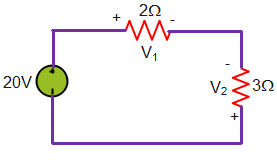What is the value of V2?

Detailed Solution for Test: Basic Concepts of Network Theory - Question 1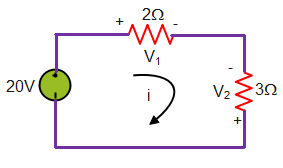From Ohm’s law,

V2= -3i and V1=2i

Appling the KVL, -20 + V1 – V2=0 ———–(i)

Put the value on above equation and get i = 4A

Hence, V2 = -3 x 4 = -12 V

Test: Basic Concepts of Network Theory - Question 2

### For the circuit given below.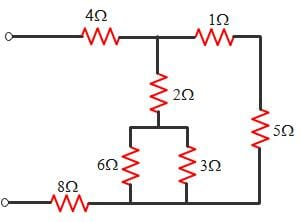What is the value of equivalent resistance?

Detailed Solution for Test: Basic Concepts of Network Theory - Question 2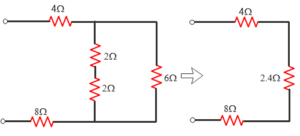Test: Basic Concepts of Network Theory - Question 3

### The circuit given below.The Thevenin resistance across the diode in the circuit is?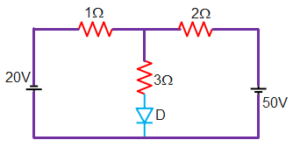The Thevenin resistance across the diode in the circuit is?

Detailed Solution for Test: Basic Concepts of Network Theory - Question 3

Diode is non-linear elements is removed.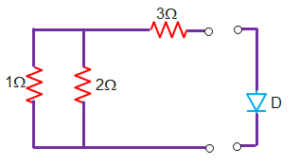After shorting the voltage source the redrawn circuit is

Thevenin resistance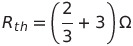= 11/3 ohm

Test: Basic Concepts of Network Theory - Question 4

Consider the following circuit.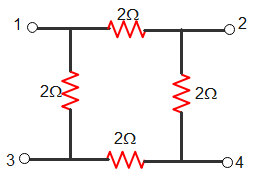What is the value of V24 / V13 of given circuit?

Detailed Solution for Test: Basic Concepts of Network Theory - Question 4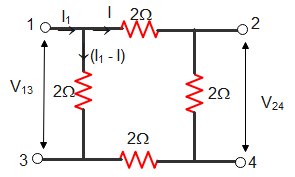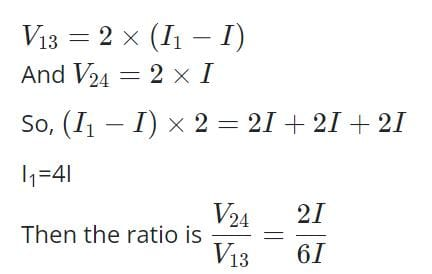Test: Basic Concepts of Network Theory - Question 5

Find the value of i.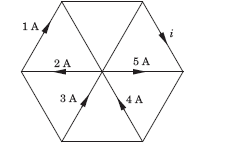Detailed Solution for Test: Basic Concepts of Network Theory - Question 5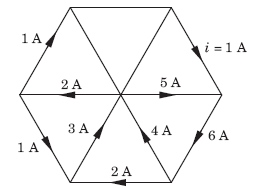• Based on kirchoffs current law, we know that incoming currents at a node is equals to outgoing currents.
• So, based on this 4 and 3 will get canceled with 5 and 2.
• So, finally i = 1.
Test: Basic Concepts of Network Theory - Question 6

In the circuit given, a charge of 600 C is delivered to the 100 V source in a 1 minute. The value of v1 must be: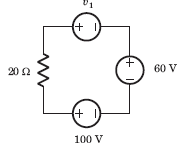Detailed Solution for Test: Basic Concepts of Network Theory - Question 6
• In order for 600 C charge to be delivered to the 100 V source, the current must be anticlockwise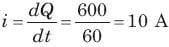• Applying KVL we get:
v1 + 60 - 100 = 10 x 20
v1 = 240 V
Test: Basic Concepts of Network Theory - Question 7

In the circuit of the fig the value of the voltage source E is: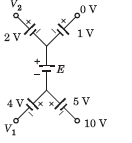Detailed Solution for Test: Basic Concepts of Network Theory - Question 7

Going from 10 V to 0 V: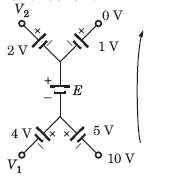• 10 + 5 + E + 1 = 0
• E = -16V
• Minus sign indicates that the polarity of battery should be reversed.
Test: Basic Concepts of Network Theory - Question 8

Consider the circuit graph shown in fig. Each branch of circuit graph represent a circuit element. The value of voltage v1 is: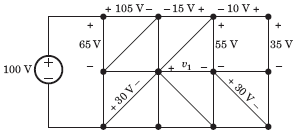Detailed Solution for Test: Basic Concepts of Network Theory - Question 8
• 100 = 65 + v2
⇒ v2 = 35 V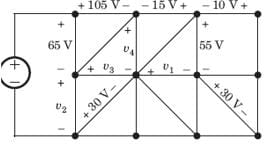• v3 - 30 = v2
⇒ v3 = 65 V
• 105 + v4 - v- 65 = 0
⇒ v4 = 25 V
• v4 + 15 - 55 + v1 = 0
⇒ v1 = 15 V
Test: Basic Concepts of Network Theory - Question 9

What quantity of charge must be delivered by a battery with a Potential Difference of 110 V to do 660J of Work?

Detailed Solution for Test: Basic Concepts of Network Theory - Question 9

► V = W / Q
► Q = W / V = 660 / 110 = 6 C

Test: Basic Concepts of Network Theory - Question 10

Find the value of R1.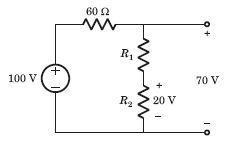Detailed Solution for Test: Basic Concepts of Network Theory - Question 10
• Voltage across 60 Ω resistor = 30 V
• Current = 30 / 60 = 0.5 A
• Voltage across R1 is = (70 - 20) V = 50 V
• R1 = 50 / 0.5 = 100 Ω
Test: Basic Concepts of Network Theory - Question 11

Twelve 6 Ω resistor are used as edge to form a cube.The resistance between two diagonally opposite corner of the cube is

Detailed Solution for Test: Basic Concepts of Network Theory - Question 11

The current i will be distributed in the cube branches symmetrically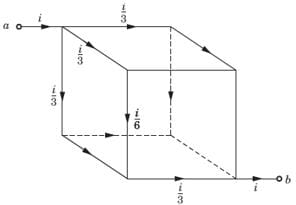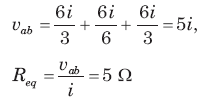Test: Basic Concepts of Network Theory - Question 12

Find the value of v1.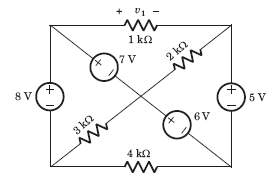Detailed Solution for Test: Basic Concepts of Network Theory - Question 12

If we go from +ve side of 1 kΩ through 7 V, 6 V and 5 V
We get, v1 = 7 + 6 - 5 = 8 V

Test: Basic Concepts of Network Theory - Question 13

The voltage vo in fig is always equal to: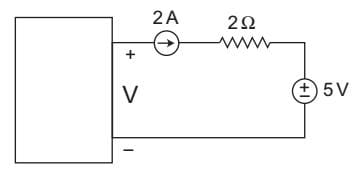Detailed Solution for Test: Basic Concepts of Network Theory - Question 13

From above figure
V – 4 – 5 = 0
or
V = 9 V
Hence alternative (D) is the correct choice.

Test: Basic Concepts of Network Theory - Question 14

In a AC circuit, resistive and total impedance are 10 and 20 ohms respectively. What is the phase angle difference between the voltage and current?

Detailed Solution for Test: Basic Concepts of Network Theory - Question 14
• Cosɸ = R/Z; where R= 10 ohms and Z = 20 ohms
• Cosɸ = 1/2 means phase angle is 60 degree.
Test: Basic Concepts of Network Theory - Question 15

The quantity of a charge that will be transferred by a current flow of 10 A over 1 hour period is _________ ?

Detailed Solution for Test: Basic Concepts of Network Theory - Question 15

We know that,
I=Q / t or Q = I x t = 10 x 1 hours
{since unit of current is Cs-1, therefore time should be in seconds}
∴ Q = 10 x 60 x 60
= 36000 C
= 3.6 x 10C

Test: Basic Concepts of Network Theory - Question 16

A capacitor is charged by a constant current of 2 mA and results in a voltage increase of 12 V in a 10 sec interval. The value of capacitance is:

Detailed Solution for Test: Basic Concepts of Network Theory - Question 16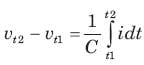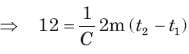12 * C  = 2 m x 10
C = 1.67 mF
Hence, Capacitance is equal to 1.67 mF.

Test: Basic Concepts of Network Theory - Question 17

The energy required to charge a 10 μF capacitor to 100 V is:

Detailed Solution for Test: Basic Concepts of Network Theory - Question 17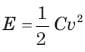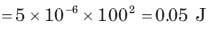Test: Basic Concepts of Network Theory - Question 18

The current in a 100 μF capacitor is shown in fig. If capacitor is initially uncharged, then the waveform for the voltage across it is: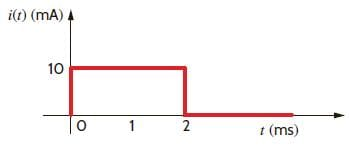Detailed Solution for Test: Basic Concepts of Network Theory - Question 18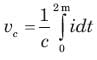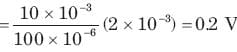This 0.2 V increases linearly from 0 to 0.2 V. Then current is zero. So capacitor hold this voltage.

Test: Basic Concepts of Network Theory - Question 19

The voltage across a 100 μF capacitor is shown in fig. The waveform for the current in the capacitor is: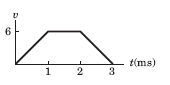Detailed Solution for Test: Basic Concepts of Network Theory - Question 19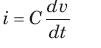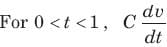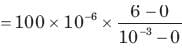= 600 mA
For 1 ms < t < 2 ms,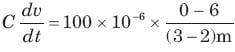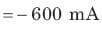Test: Basic Concepts of Network Theory - Question 20

The waveform for the current in a 200 μF capacitor is shown in fig. The waveform for the capacitor voltage is: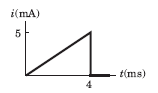Detailed Solution for Test: Basic Concepts of Network Theory - Question 20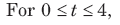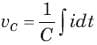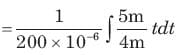= 3125 t2
At t = 4 ms, vc = 0.05 V
It will be parabolic path at t = 0
t-axis will be tangent.

## GATE Electrical Engineering (EE) 2023 Mock Test Series

21 docs|271 tests
 Use Code STAYHOME200 and get INR 200 additional OFF Use Coupon Code
Information about Test: Basic Concepts of Network Theory Page
In this test you can find the Exam questions for Test: Basic Concepts of Network Theory solved & explained in the simplest way possible. Besides giving Questions and answers for Test: Basic Concepts of Network Theory, EduRev gives you an ample number of Online tests for practice

## GATE Electrical Engineering (EE) 2023 Mock Test Series

21 docs|271 tests